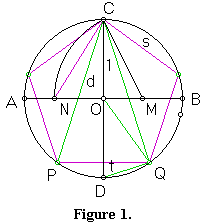# Inscribing a regular pentagon in a circle - and proving it

### Scott E. Brodie

Constructions by straight-edge and compass of several regular polygons - the equilateral triangle, square, hexagon, and octagon, are familiar. Indeed, the construction of an equilateral triangle is the very first proposition in Euclid's Elements (I.1). Less well known is the surprisingly simple construction of a regular pentagon by the same methods. The construction and its verification give another illustration of how the Pythagorean Theorem is woven into the structure of geometry, even though there are no right angles in a pentagon. (A regular pentagon can also be modeled by flattening an overhand knot tied from a strip of uniform width.)Start with a circle of radius $1$ (Figure 1).

Construct perpendicular diameters $AB$ and $CD.$ Bisect the radius $OB$ at $M.$ With center $M$ and radius $MC,$ draw an arc intersecting radius $OA$ at $N.$ I claim that $CN$ is the side of the regular pentagon inscribed in the circle.

To verify the construction, look first at the "star pentagram", a regular pentagon containing a five-pointed star inscribed within it (Figure 2). Consider the two diagonals radiating from a single vertex. These two diagonals enclose one side of the pentagon, forming an isosceles triangle (such as $CPQ$ in Figure 2).The Greek geometers realized that this triangle demonstrates a property of "self-similarity" comparable to that of the better-known "golden rectangle": since the ray $PR$ bisects angle $CPQ,$ triangle $PQR$ is similar to the original triangle $CPQ.$ Thus $CP/PQ = PQ/RQ = PQ/(CP - PQ),$ or $PQ^{2} = CP(CP-PQ).$ Setting $CP = \phi \cdot PQ,$ we have $1 = \phi \cdot (\phi - 1) = \phi ^{2} - \phi .$ The quadratic formula yields $\displaystyle\frac{1+\sqrt{5}}{2}.$ The quantity \phi is known as the "golden ratio", and appears in disparate contexts in geometry and number theory.

Now return to the original construction (Figure 1). To compute the length of segment $CN,$ use the Pythagorean Theorem twice: $CM^{2} = 1+ 1/4$ but $ON = CM - 1/2;$ so $CN^{2} = 1 + (\sqrt{5/4}-1/2)^{2} = 5/2 - \sqrt{5}/2.$

On the other hand, let $s$ be the length of the side of the inscribed pentagon, $d$ the length of a diagonal, and $t$ the length of the segment $DQ$ in the figure (the side of a regular decagon inscribed in the same circle). We have $d = \phi s.$

But triangle $ODQ$ is similar to triangle $CPQ,$ so we have likewise $1 = \phi t.$

So far, we have two equations in the three variables $d,$ $s,$ and $t.$ Fortunately, the Pythagorean Theorem gives us a third equation: triangle $CDQ$ is inscribed in a semicircle, so angle $DQC$ is right, and $t^{2} + d^{2} = 4.$

Then $s = d / \phi = \phi ^{-1}\sqrt{4 - 1/\phi^{2}} = \sqrt{5/2-\sqrt{5}/2},$ QED.

Elegant as this construction is, this is not how it appears in Euclid's Elements. The construction is commonly attributed to another Greek mathematican Ptolemy who lived some 400 years later. Euclid's procedure (IV.11) is as follows: begin with a line segment divided by a point into two segments whose ratio is \phi (II.11). (Note how this construction bears a strong resemblance to the construction of the pentagon given above.) Next, construct an isosceles triangle with sides proportional to $\phi,$ $\phi,$ and $1$ (IV.10). Euclid notes that in such a triangle, the base angles are double the vertex angle. Inscribe a triangle similar to this one in a given circle (IV.2) . Now bisect the base angles of the inscribed triangle. The intersections of the bisecting rays with the circle, together with the vertices of the inscribed triangle, determine the desired pentagon.

(This construction is easily implementable by paper folding. Also, there is also an elegant 19th century Japanese construction of the regular pentagon.)### Fibonacci Numbers